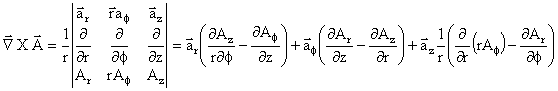# Curl of a Vector

In vector calculus, the curl (or rotor) is a vector operator that describes the rotation of a vector field. At every point in the field, the curl is represented by a vector. The attributes of this vector (length and direction) characterize the rotation at that point.

The direction of the curl is the axis of rotation, as determined by the right-hand rule, and the magnitude of the curl is the magnitude of rotation. If the vector field represents the flow velocity of a moving fluid, then the curl is the circulation density of the fluid. A vector field whose curl is zero is called irrational. The curl is a form of differentiation for vector fields. The corresponding form of the fundamental theorem of calculus is Stokes' theorem, which relates the surface integral of the curl of a vector field to the line integral of the vector field around the boundary curve. - Wikipedia

"Ñ" is a vector and is pronounced "del." The vector function is A(x,y,z), A(r,Φ,z), or A(r,θ,Φ)

 Cartesian CoordinatesCylindrical CoordinatesSpherical CoordinatesAbout RF CafeCopyright: 1996 - 2024Webmaster:    Kirt Blattenberger,    BSEE - KB3UON RF Cafe began life in 1996 as "RF Tools" in an AOL screen name web space totaling 2 MB. Its primary purpose was to provide me with ready access to commonly needed formulas and reference material while performing my work as an RF system and circuit design engineer. The Internet was still largely an unknown entity at the time and not much was available in the form of WYSIWYG ... All trademarks, copyrights, patents, and other rights of ownership to images and text used on the RF Cafe website are hereby acknowledged. My Hobby Website:  AirplanesAndRockets.com